# Vacuum Cleaner

The objective of this practice is to implement the logic of a navigation algorithm for an autonomous vacuum. The main objective will be to cover the largest area of ​​a house using the programmed algorithm.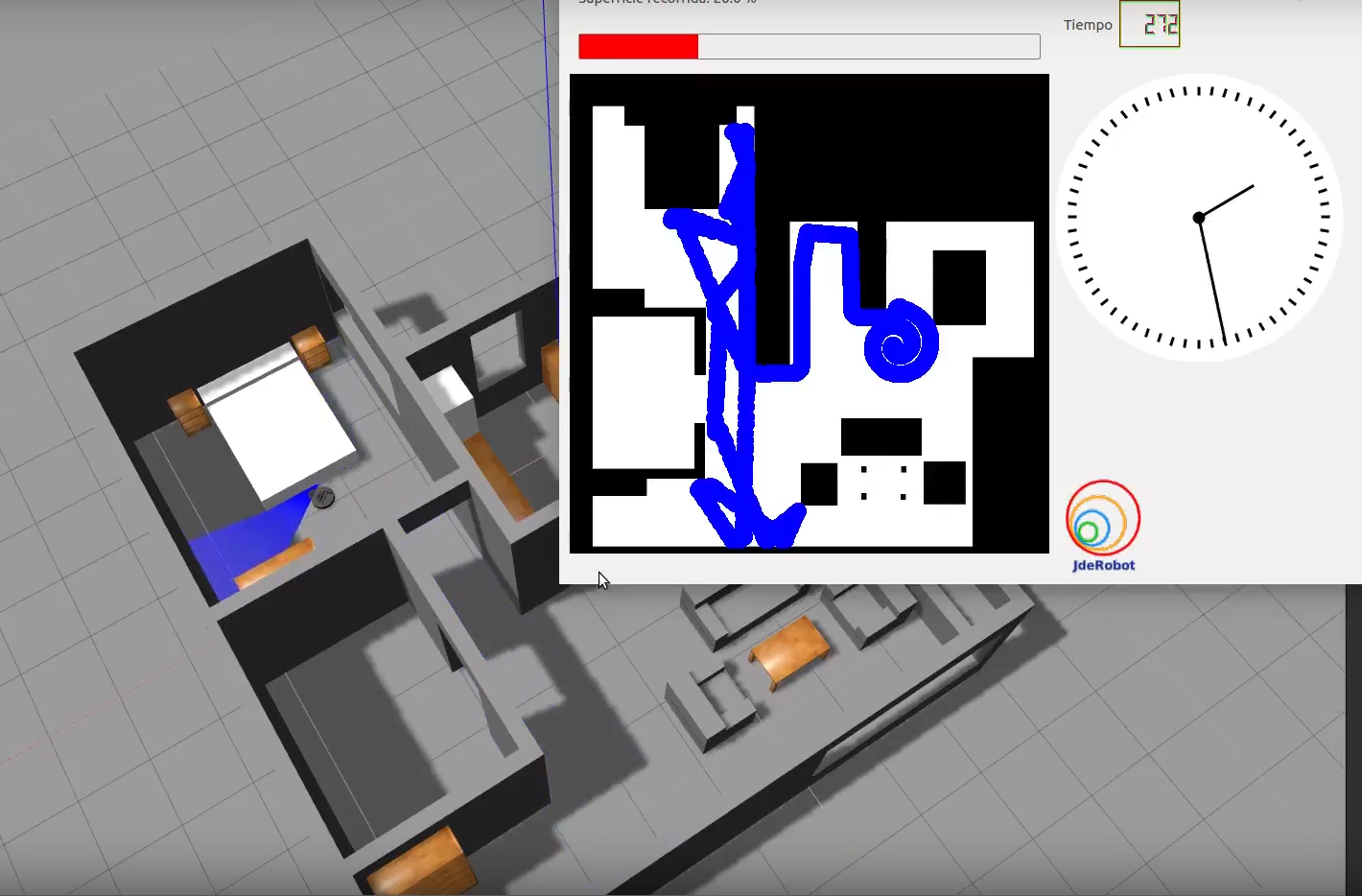## Installation

Install the General Infrastructure of the JdeRobot Robotics Academy.

Enable Kobuki_msgs:

sudo apt-get install ros-melodic-kobuki-msgs


## How to run

1. Execution without watching the world:
roslaunch vacuum_cleaner.launch

2. Execution of the practice and the user interface:
python2 vacuumCleaner.py vacuumCleaner_conf.yml

3. Execution of the automatic evaluator:
python2 referee.py referee.yml


To simplify the closure of the environment, simply close the VacuumCleaner window(s). Ctrl + C will give problems.

## How to do the practice

To carry out the practice, you must edit the MyAlgorithm.py file and insert the control logic into it.

## Where to insert the code

MyAlgorithm.py

print ('Execute')

# TODO

print self.pose3d.getPose3d().x
print self.pose3d.getPose3d().y
# Vacuum's yaw
yaw = self.pose3d.getPose3d().yaw

# EXAMPLE OF HOW TO SEND INFORMATION TO THE ROBOT ACTUATORS
vel = CMDVel()
myW=x*(self.motors.getMaxW())
myV=-y*(self.motors.getMaxV())
vel.vx = myV
vel.az = myW
self.motors.sendVelocities(vel)
# OR
self.motors.sendAZ(vel.az)
self.motors.sendV(vel.vx)


## API

• self.pose3d.getPose3d().yaw - to get the orientation of the robot
• self.bumper.getBumperData().state - to establish if the robot has crashed or not. Returns a 1 if the robot collides and a 0 if it has not crashed.
• self.bumper.getBumperData().bumper - If the robot has crashed, it turns to 1 when the crash occurs at the center of the robot, 0 when it occurs at its left and 2 if the collision is at its right.
• laser.getLaserData() - It allows to obtain the data of the laser sensor, which consists of 180 pairs of values ​​(0-180º, distance in millimeters).
• self.motors.sendVelocities(vel) - to set linear and angular velocity. Object ‘vel’ must be a CMDVel():
• vel = CMDVel()
• vel.vx = v - linear velocity
• vel.az = w - angular velocity

For this example, it is necessary to ensure that the vacuum cleaner covers the highest possible percentage of the house. The application of the automatic evaluator (referee) will measure the percentage traveled, and based on this percentage, will perform the qualification of the solution algorithm.

### Types conversion

• Laser
laser_data = self.laser.getLaserData()

def parse_laser_data(laser_data):
laser = []
for i in range(180):
dist = laser_data.values[i]
laser += [(dist, angle)]
return laser

def laser_vector(laser):
laser_vectorized = []
for d,a in laser:
# (4.2.1) laser into GUI reference system
x = d * math.cos(a) * -1
y = d * math.sin(a) * -1
v = (x,y)
laser_vectorized += [v]

laser_mean = np.mean(laser_vectorized, axis=0)
return laser_mean


## Theory

Implementation of navigation algorithms for an autonomous vacuum is the basic requirement for this exercise. The main objective is to cover the largest area of a house. First, let us understand what is Coverage Algorithms.

### Coverage Algorithms

Coverage Path Planning is an important area of research in Path Planning for robotics, which involves finding a path that passes through every reachable position in its environment. In this exercise, We are using a very basic coverage algorithm called Random Exploration.## Analyzing Coverage Algorithms

### Classification

Coverage algorithms are divided into two categories.

• Offline coverage use fixed information and the environment is known in advance. Genetic Algorithms, Neural Networks, Cellular Decomposition, Spanning Trees are some examples to name a few.

• Online Coverage

Uses real-time measurements and decisions to cover the entire area. The Sensor-based approach is included in this category.

### Base Movement

The problem of coverage involves two standard basic motions, which are used as a base for other complex coverage algorithms.

• Spiral Motion

The robot follows an increasing circle/square pattern.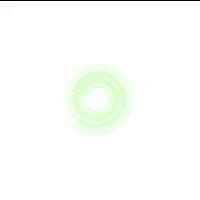• Boustrophedon Motion

The robot follows an S-shaped pattern.### Analysis of Coverage Algorithms

Any coverage algorithm is analyzed using the given criterion.

• Environment Decomposition This involves dividing the area into smaller parts.

• Sweep Direction

This influences the optimality of generated paths for each sub-region by adjusting the duration, speed, and direction of each sweep.

• Optimal Backtracking

This involves the plan to move from one small subregion to another. The coverage is said to be complete when there is no point left to backtrack.

### Supplements

Usually, coverage algorithms generate a linear, piecewise path composed of straight lines and sharp turns. This path is difficult for other autonomous drones like Underwater Vehicles, Aerial Vehicles and some Ground Vehicles difficult to follow. Path Smoothening is applied to these paths to effectively implement the algorithm.

## Hints

Simple hints provided to help you solve the vacuum_cleaner exercise. Please note that the full solution has not been provided.

### Random Angle Generation

The most important task is the generation of a random angle. There are 2 ways to achieve it.

• Random Duration: By keeping the angular_velocity fixed, the duration of the turn can be randomized, in order to point the robot towards a random direction.

• Random Angle: This method requires calculation. We generate a random angle and then turn towards it. Approximately an angular speed of 3 turns the robot by 90 degrees.

Among both the methods, Random Duration would be a preferable one as the Random Angle requires precision, which requires PID to be achieved successfully.

Also, in order to acheive better precision it is preferable to use rospy.sleep() in place of time.sleep().

### Dash Movement

Once the direction has been decided, we move in that direction. This is the simplest part, we have to send velocity command to the robot, until a collision is detected.

A word of caution though, whenever we have a change of state, we have to give a sleep duration to the robot to give it time to reset the commands given to it. Illustrations section describes a visual representation.

### Spiral Movement

Using the physical formula $v = r·\omega$ (See references for more details). In order to increase $r$, we can either increase $v$ or decrease $\omega$, while keeping the other parameter constant. Experimentally, increasing $v$ has a better effect than decreasing $\omega$. Refer to illustrations.

### Analysis

Being such a simple algorithm, it is not expected to work all the time. The maximum accuracy we got was 80% and that too only once!

### Illustrations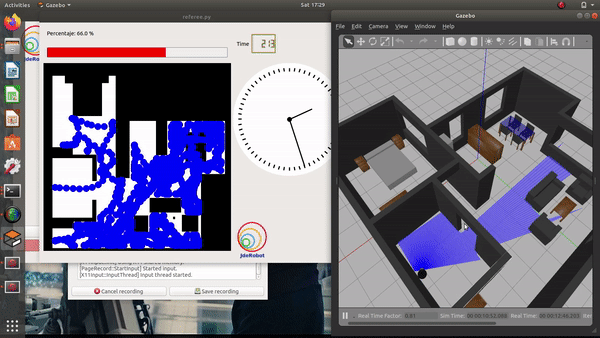Without applying a sleep duration the previous rotation command still has effect on the go straight command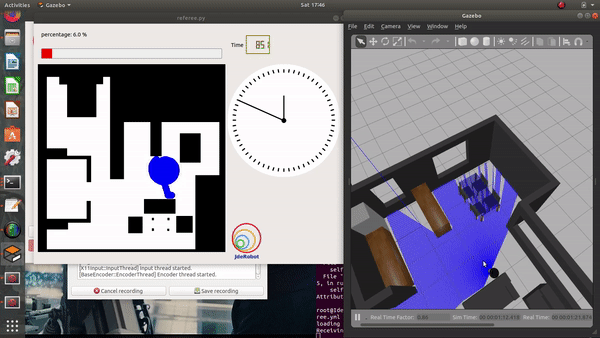After applying a duration, we get straight direction movement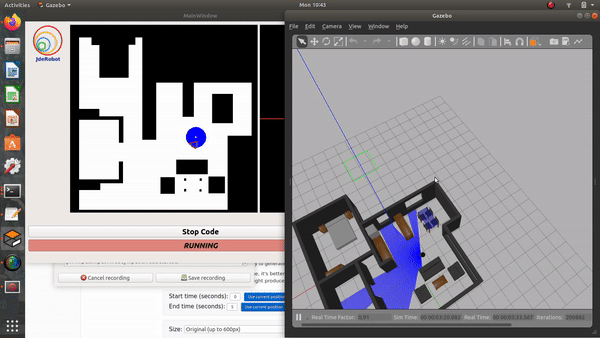Effect of reducing $\omega$ to generate spiral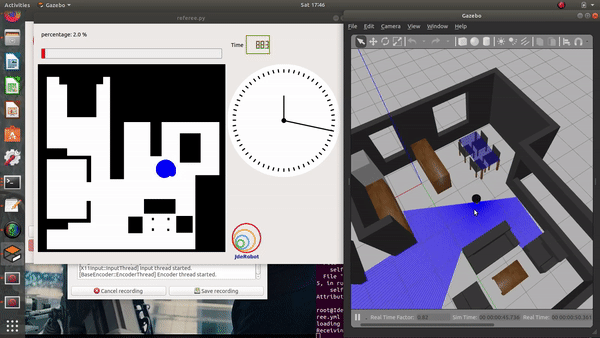Effect of increasing $v$ to generate spiral

One possible solution is to implement the logic of a navigation algorithm for an autonomous vacuum without autolocation.